# APOGEE Spectra with Censored Bayesian NN - ApogeeBCNNCensored

class astroNN.models.apogee_models.ApogeeBCNNCensored(lr=0.0005, dropout_rate=0.3)[source]

Class for Bayesian censored convolutional neural network for stellar spectra analysis [specifically APOGEE DR14 spectra only]

History:

2018-May-27 - Written - Henry Leung (University of Toronto)

digraph inheritanceccaffbb238 { bgcolor=transparent; dpi=144; rankdir=LR; size="8.0, 12.0"; "ABC" [URL="https://docs.python.org/3/library/abc.html#abc.ABC",dpi=144,fillcolor=white,fontname="Vera Sans, DejaVu Sans, Liberation Sans, Arial, Helvetica, sans",fontsize=10,height=0.25,shape=box,style="setlinewidth(0.5),filled",target="_top",tooltip="Helper class that provides a standard way to create an ABC using"]; "ApogeeBCNNCensored" [URL="#astroNN.models.apogee_models.ApogeeBCNNCensored",dpi=144,fillcolor=white,fontname="Vera Sans, DejaVu Sans, Liberation Sans, Arial, Helvetica, sans",fontsize=10,height=0.25,shape=box,style="setlinewidth(0.5),filled",target="_top",tooltip="Class for Bayesian censored convolutional neural network for stellar spectra analysis [specifically APOGEE"]; "BayesianCNNBase" -> "ApogeeBCNNCensored" [arrowsize=0.5,style="setlinewidth(0.5)"]; "BayesianCNNBase" [URL="basic_usage.html#astroNN.models.base_bayesian_cnn.BayesianCNNBase",dpi=144,fillcolor=white,fontname="Vera Sans, DejaVu Sans, Liberation Sans, Arial, Helvetica, sans",fontsize=10,height=0.25,shape=box,style="setlinewidth(0.5),filled",target="_top",tooltip="Top-level class for a Bayesian convolutional neural network"]; "NeuralNetMaster" -> "BayesianCNNBase" [arrowsize=0.5,style="setlinewidth(0.5)"]; "ABC" -> "BayesianCNNBase" [arrowsize=0.5,style="setlinewidth(0.5)"]; "NeuralNetMaster" [URL="basic_usage.html#astroNN.models.base_master_nn.NeuralNetMaster",dpi=144,fillcolor=white,fontname="Vera Sans, DejaVu Sans, Liberation Sans, Arial, Helvetica, sans",fontsize=10,height=0.25,shape=box,style="setlinewidth(0.5),filled",target="_top",tooltip="Top-level class for an astroNN neural network"]; "ABC" -> "NeuralNetMaster" [arrowsize=0.5,style="setlinewidth(0.5)"]; }

ApogeeBCNNCensored can only be used with Apogee spectra with 7,514 pixels

from astroNN.models import ApogeeBCNNCensored

# Load the train data from dataset first, x_train is spectra and y_train will be ASPCAP labels
loader.target = ['teff', 'logg', 'C', 'C1', 'N', 'O', 'Na', 'Mg', 'Al', 'Si', 'P', 'S', 'K',
'Ca', 'Ti', 'Ti2', 'V', 'Cr', 'Mn', 'Fe','Co', 'Ni']

# And then create an instance of Apogee Censored Bayesian Convolutional Neural Network class
bcnncensored_net = ApogeeBCNNCensored()

# Set max_epochs to 10 for a quick result. You should train more epochs normally, especially with dropout
bcnncensored_net.max_epochs = 10
bcnncensored_net.train(x_train, y_train, x_err, y_err)


Here is a list of parameter you can set but you can also not set them to use default

ApogeeBCNNCensored.batch_size = 64
ApogeeBCNNCensored.initializer = 'he_normal'
ApogeeBCNNCensored.activation = 'relu'
ApogeeBCNNCensored.num_filters = [2, 4]
ApogeeBCNNCensored.filter_len = 8
ApogeeBCNNCensored.pool_length = 4
# number of neurone for [old_bcnn_1, old_bcnn_2, aspcap_1, aspcap_2, hidden]
ApogeeBCNNCensored.num_hidden = [128, 64, 32, 8, 2]
ApogeeBCNNCensored.max_epochs = 50
ApogeeBCNNCensored.lr = 0.005
ApogeeBCNNCensored.reduce_lr_epsilon = 0.00005
ApogeeBCNNCensored.reduce_lr_min = 0.0000000001
ApogeeBCNNCensored.reduce_lr_patience = 10
ApogeeBCNNCensored.target = 'all'
ApogeeBCNNCensored.l2 = 5e-9
ApogeeBCNNCensored.dropout_rate = 0.2
ApogeeBCNNCensored.length_scale = 0.1  # prior length scale
ApogeeBCNNCensored.input_norm_mode = 3
ApogeeBCNNCensored.labels_norm_mode = 2


Note

You can disable astroNN data normalization via ApogeeBCNNCensored.input_norm_mode=0 as well as ApogeeBCNNCensored.labels_norm_mode=0 and do normalization yourself. But make sure you don’t normalize labels with MAGIC_NUMBER (missing labels).

After the training, you can use bcnncensored_net in this case and call test method to test the neural network on test data. Or you can load the folder by

from astroNN.models import load_folder

# Load the test data from dataset, x_test is spectra and y_test will be ASPCAP labels
loader2.target = ['teff', 'logg', 'C', 'C1', 'N', 'O', 'Na', 'Mg', 'Al', 'Si', 'P', 'S', 'K',
'Ca', 'Ti', 'Ti2', 'V', 'Cr', 'Mn', 'Fe','Co', 'Ni']

# pred contains denormalized result aka. ASPCAP labels prediction in this case
# pred_std is a list of uncertainty
# pred_std['total'] is the total uncertainty (standard derivation) which is the sum of all the uncertainty
# pred_std['predictive'] is the predictive uncertainty predicted by bayesian neural net
# pred_std['model'] is the model uncertainty from dropout variational inference
pred, pred_std = bcnncensored_net.test(x_test)

bcnncensored_net.aspcap_residue_plot(pred, y_test, pred_std['total'])


You can calculate jacobian which represents the output derivative to the input and see where those output is sensitive to in inputs.

# Calculate jacobian first
jacobian_array = bcnncensored_net.jacobian(x_test, mean_output=True)

# Plot the graphs
bcnncensored_net.jacobian_aspcap(jacobian=jacobian_array, dr=14)


## Architecture

The architecture of this neural network is as follow.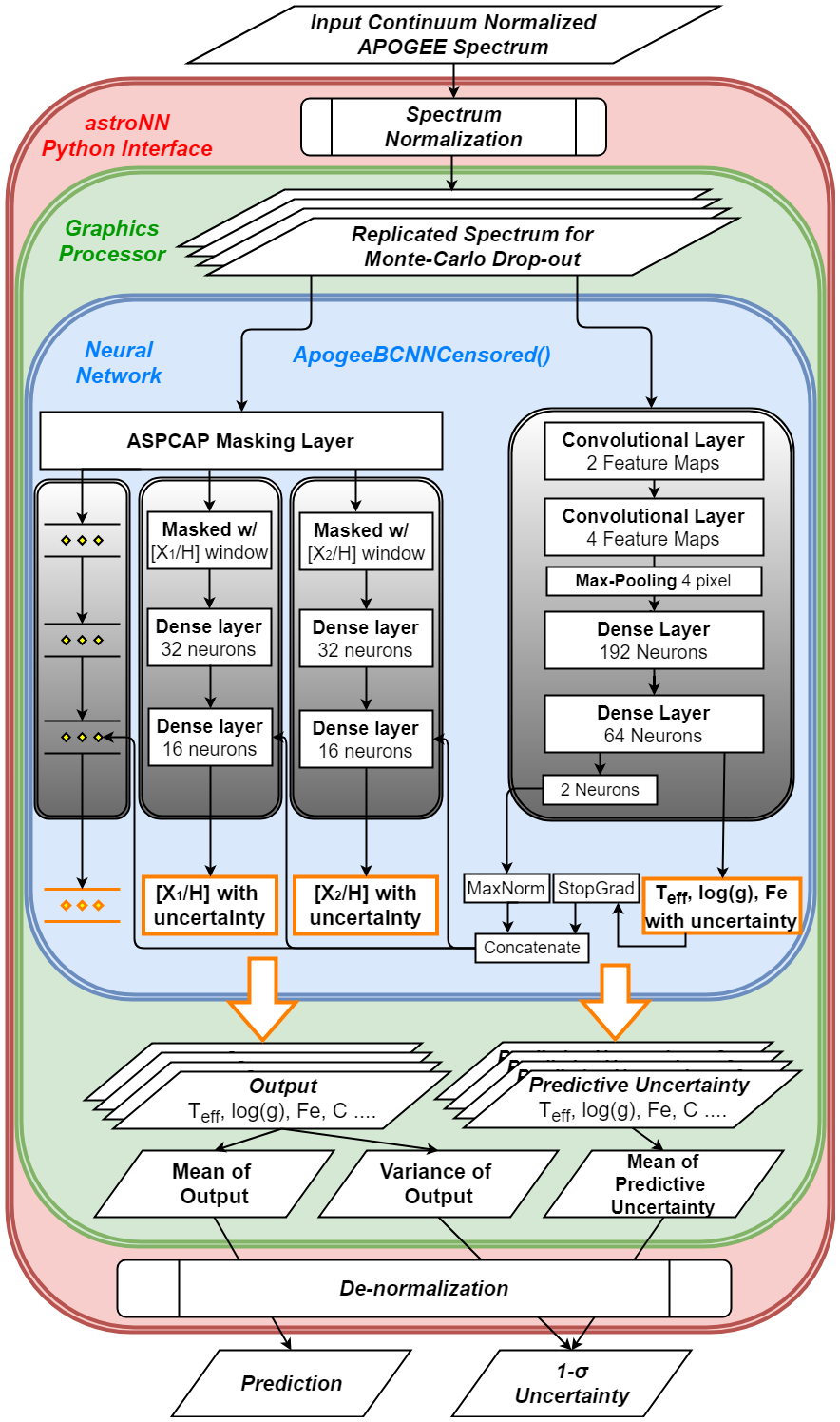## Why Censored NN for APOGEE Spectra analysis?

Internal model identifier for the author: astroNN_0529_run010

It caught our attention that ApogeeBCNN neural network found no spread in $$[Al/H]$$ in $$M13$$ globular cluster (Literature of showing a spread in $$[Al/H]$$: https://arxiv.org/pdf/1501.05127.pdf) and it may imply a problem in ApogeeBCNN that it found strongly correlation between elements but not actually measuring individually.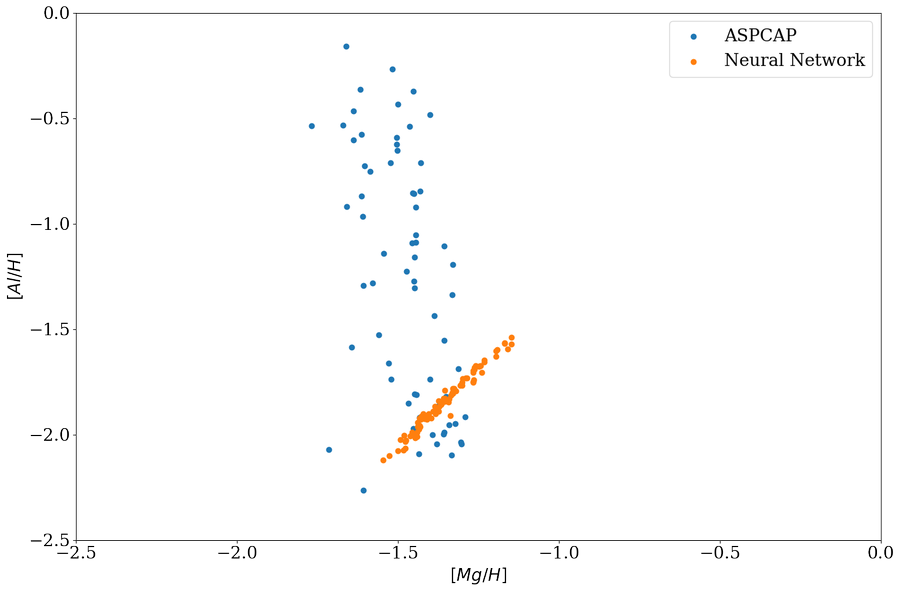It becomes clear when we plot the training set $$[Al/H]$$ vs $$[Mg/H]$$ as follow, $$[Al/H]$$ and $$[Mg/H]$$ are strongly correlated and ApogeeBCNN is just measuring $$[Al/H]$$ as some kind of $$[Mg/H]$$ and fooled in $$M13$$ because $$M13$$ has a spread in $$[Al/H]$$ but not $$[Mg/H]$$, in other word, the region in $$[Mg/H, Al/H]$$ parameter space of $$M13$$ is not covered by training set.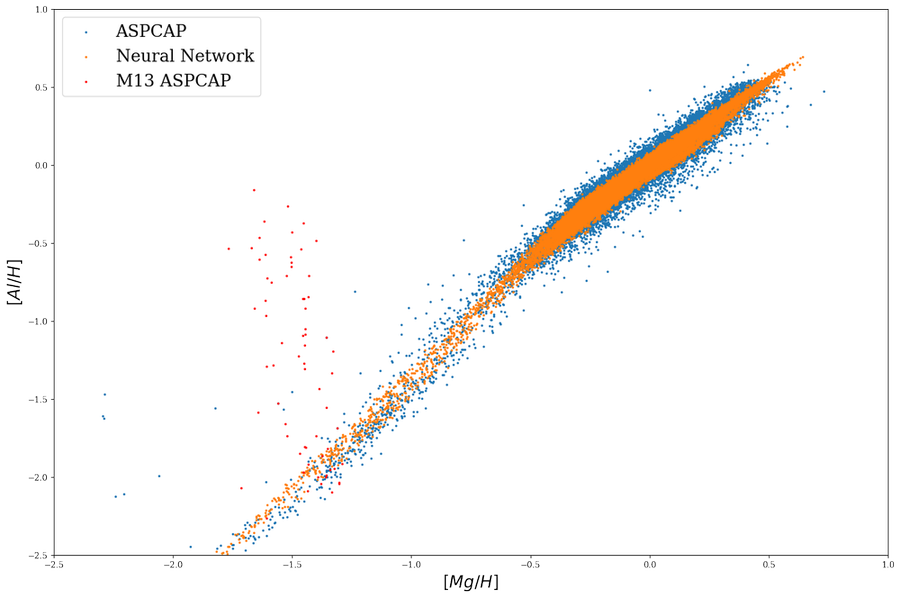So Censored Neural Net is proposed to solve the issue by encouraging neural network to look at the ASPCAP window regions.

And it seems like it solved the issue and now neural network show a spread in $$[Al/H]$$ but not $$[Mg/H]$$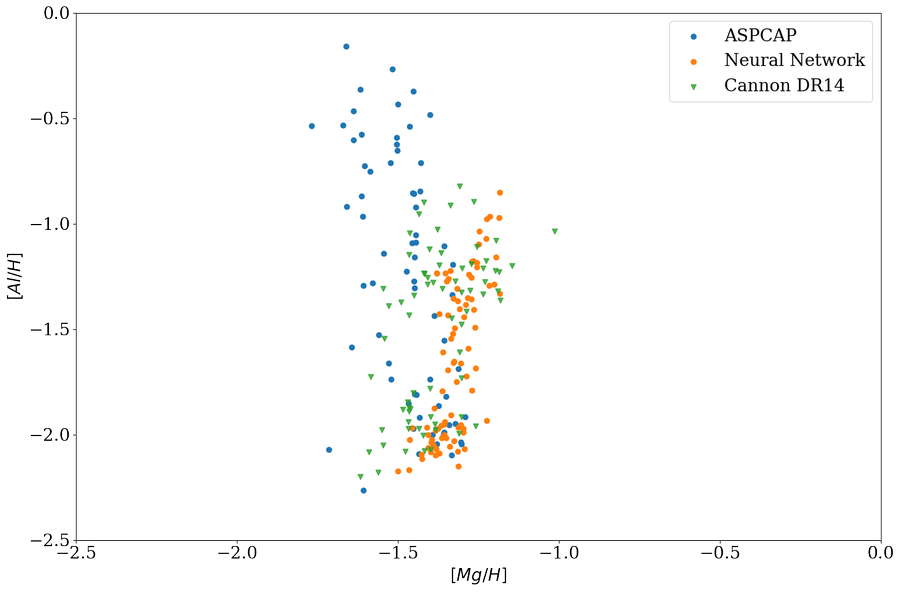with this censored neural network and plot the training set, indeed it shows a little more spread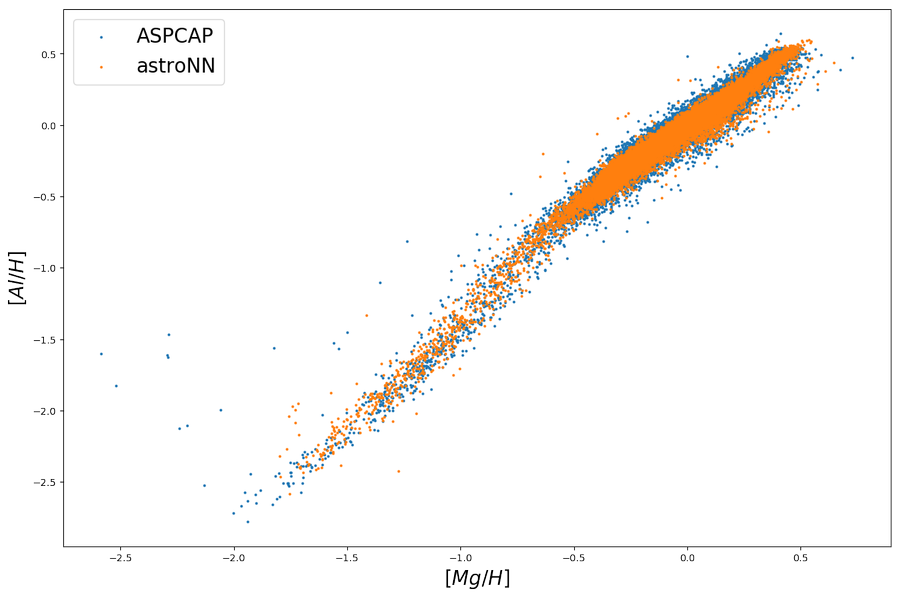## ASPCAP Labels Prediction

Internal model identifier for the author: astroNN_0529_run010

Training set and Testing set is exactly the same as APOGEE Spectra with Bayesian Neural Net - ApogeeBCNN

Training set (30067 spectra + separate 3340 validation spectra): Starflag=0 and ASPCAPflag=0, 4000<Teff<5500, 200<SNR

Testing set (97723 spectra): Individual Visit of the training spectra, median SNR is around SNR~100

Using astroNN.models.ApogeeBCNNCensored with default hyperparameter

Ground Truth is ASPCAP labels.

Median of residue

Al

-0.002

0.047

C

0.000

0.033

C1

0.000

0.044

Ca

0.001

0.024

Co

-0.002

0.072

Cr

-0.006

0.033

Fe

-0.003

0.019

K

-0.001

0.036

Log(g)

0.006

0.049

Mg

-0.002

0.021

Mn

-0.004

0.032

N

-0.004

0.035

Na

-0.014

0.118

Ni

-0.003

0.023

O

0.001

0.033

P

0.001

0.100

S

0.000

0.048

Si

-0.002

0.024

Teff

2.310

23.296

Ti

-0.001

0.035

Ti2

-0.006

0.090

V

-0.002

0.067